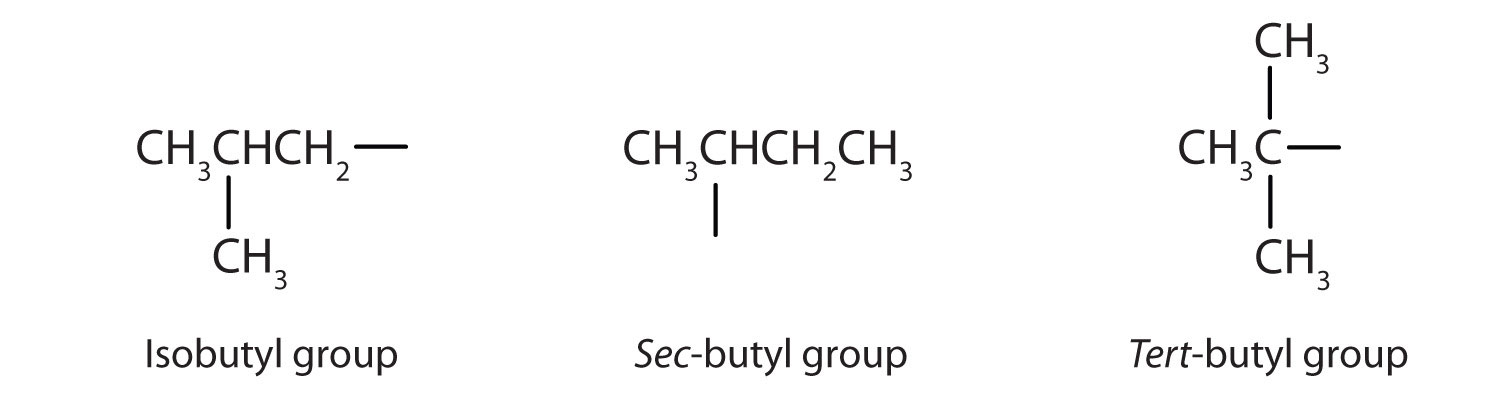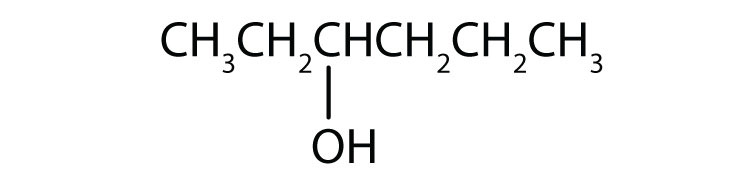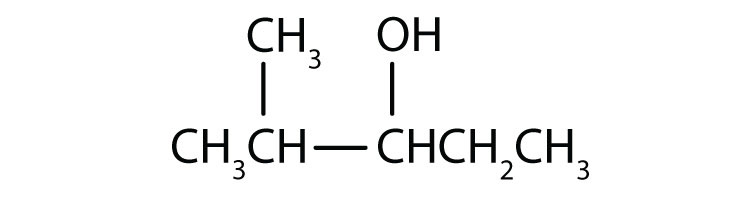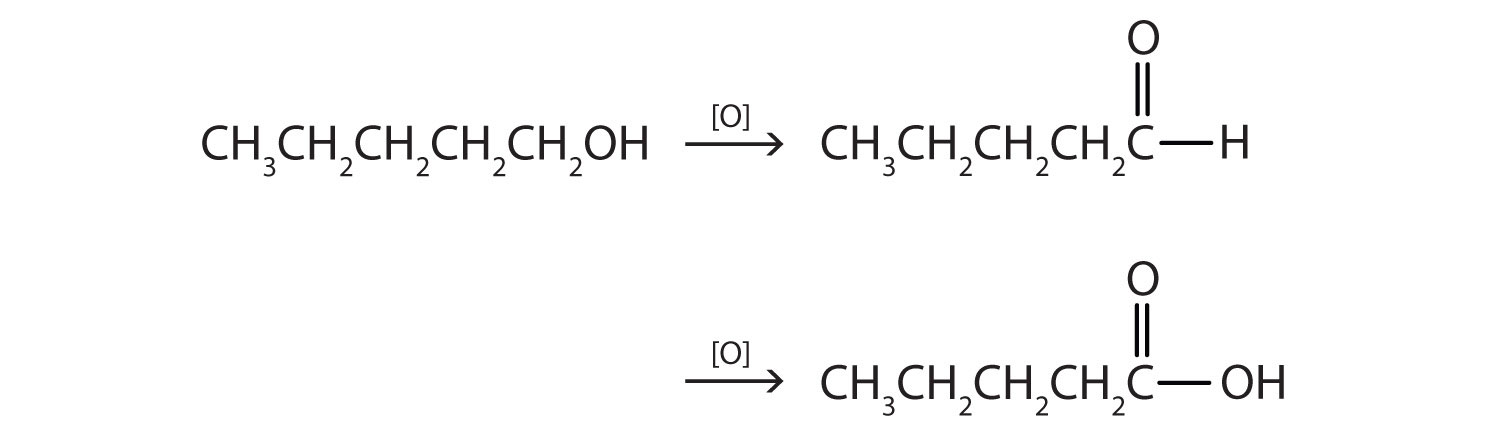# 10.9: Alcohols

Learning Objectives

• Identify the general structure for an alcohol.
• Identify the structural feature that classifies alcohols as primary, secondary, or tertiary.
• Name alcohols with both common names and IUPAC names

An alcohol is an organic compound with a hydroxyl (OH) functional group on an aliphatic carbon atom. Because OH is the functional group of all alcohols, we often represent alcohols by the general formula ROH, where R is an alkyl group. Alcohols are common in nature. Most people are familiar with ethyl alcohol (ethanol), the active ingredient in alcoholic beverages, but this compound is only one of a family of organic compounds known as alcohols. The family also includes such familiar substances as cholesterol and the carbohydrates. Methanol (CH3OH) and ethanol (CH3CH2OH) are the first two members of the homologous series of alcohols.

## Nomenclature of Alcohols

Alcohols with one to four carbon atoms are frequently called by common names, in which the name of the alkyl group is followed by the word alcohol:According to the International Union of Pure and Applied Chemistry (IUPAC), alcohols are named by changing the ending of the parent alkane name to -ol. Here are some basic IUPAC rules for naming alcohols:

1. The longest continuous chain (LCC) of carbon atoms containing the OH group is taken as the parent compound—an alkane with the same number of carbon atoms. The chain is numbered from the end nearest the OH group.
2. The number that indicates the position of the OH group is prefixed to the name of the parent hydrocarbon, and the -e ending of the parent alkane is replaced by the suffix -ol. (In cyclic alcohols, the carbon atom bearing the OH group is designated C1, but the 1 is not used in the name.) Substituents are named and numbered as in alkanes.
3. If more than one OH group appears in the same molecule (polyhydroxy alcohols), suffixes such as -diol and -triol are used. In these cases, the -e ending of the parent alkane is retained.

Figure $$\PageIndex{1}$$ shows some examples of the application of these rules.Figure $$\PageIndex{1}$$: IUPAC Rules for Alcohols. The names and structures of some alcohols demonstrate the use of IUPAC rules.

Example $$\PageIndex{1}$$

Give the IUPAC name for each compound.

1.2. HOCH2CH2CH2CH2CH2OH

Solution

1. Ten carbon atoms in the LCC makes the compound a derivative of decane (rule 1), and the OH on the third carbon atom makes it a 3-decanol (rule 2).The carbon atoms are numbered from the end closest to the OH group. That fixes the two methyl (CH3) groups at the sixth and eighth positions. The name is 6,8-dimethyl-3-decanol (not 3,5-dimethyl-8-decanol).

2. Five carbon atoms in the LCC make the compound a derivative of pentane. Two OH groups on the first and fifth carbon atoms make the compound a diol and give the name 1,5-pentanediol (rule 3).Exercise $$\PageIndex{1}$$

Give the IUPAC name for each compound.

1.2.Example $$\PageIndex{2}$$

Draw the structure for each compound.

1. 2-hexanol
2. 3-methyl-2-pentanol

Solution

1. The ending -ol indicates an alcohol (the OH functional group), and the hex- stem tells us that there are six carbon atoms in the LCC. We start by drawing a chain of six carbon atoms: –C–C–C–C–C–C–.

The 2 indicates that the OH group is attached to the second carbon atom.Finally, we add enough hydrogen atoms to give each carbon atom four bonds.2. The ending -ol indicates an OH functional group, and the pent- stem tells us that there are five carbon atoms in the LCC. We start by drawing a chain of five carbon atoms: –C–C–C–C–C–

The numbers indicate that there is a methyl (CH3) group on the third carbon atom and an OH group on the second carbon atom.Exercise $$\PageIndex{2}$$

Draw the structure for each compound.

1. 3-heptanol

2. 2-methyl-3-hexanol

## Classification of Alcohols

Some of the properties of alcohols depend on the number of carbon atoms attached to the specific carbon atom that is attached to the OH group. Alcohols can be grouped into three classes on this basis.

• A primary (1°) alcohol is one in which the carbon atom (in red) with the OH group is attached to one other carbon atom (in blue). Its general formula is RCH2OH.• A secondary (2°) alcohol is one in which the carbon atom (in red) with the OH group is attached to two other carbon atoms (in blue). Its general formula is R2CHOH.• A tertiary (3°) alcohol is one in which the carbon atom (in red) with the OH group is attached to three other carbon atoms (in blue). Its general formula is R3COH.Table $$\PageIndex{1}$$ names and classifies some of the simpler alcohols. Some of the common names reflect a compound’s classification as secondary (sec-) or tertiary (tert-). These designations are not used in the IUPAC nomenclature system for alcohols. Note that there are four butyl alcohols in the table, corresponding to the four butyl groups: the butyl group (CH3CH2CH2CH2) discussed before, and three others:Table $$\PageIndex{1}$$: Classification and Nomenclature of Some Alcohols
Condensed Structural Formula Class of Alcohol Common Name IUPAC Name
CH3OH methyl alcohol methanol
CH3CH2OH primary ethyl alcohol ethanol
CH3CH2CH2OH primary propyl alcohol 1-propanol
(CH3)2CHOH secondary isopropyl alcohol 2-propanol
CH3CH2CH2CH2OH primary butyl alcohol 1-butanol
CH3CH2CHOHCH3 secondary sec-butyl alcohol 2-butanol
(CH3)2CHCH2OH primary isobutyl alcohol 2-methyl-1-propanol
(CH3)3COH tertiary tert-butyl alcohol 2-methyl-2-propanolsecondary cyclohexyl alcohol cyclohexanol

## Summary

In the IUPAC system, alcohols are named by changing the ending of the parent alkane name to -ol. Alcohols are classified according to the number of carbon atoms attached to the carbon atom that is attached to the OH group.

## Concept Review Exercises

1. Is isobutyl alcohol primary, secondary, or tertiary? Explain.2. What is the LCC in 2-ethyl-1-hexanol? What is taken as the LCC in naming the compound? Explain.

1. primary; the carbon atom bearing the OH group is attached to only one other carbon atom

2. 7 carbon atoms; the 6-atom chain includes the carbon atom bearing the OH group

## Exercises

1. Name each alcohol and classify it as primary, secondary, or tertiary.

1. CH3CH2CH2CH2CH2CH2OH
2.3.2. Name each alcohol and classify it as primary, secondary, or tertiary.

1.2.3.3. Draw the structure for each alcohol.

1. 3-hexanol
2. 3,3-dimethyl-2-butanol
3. cyclobutanol
4. Draw the structure for each alcohol.

1. cyclopentanol
2. 4-methyl-2-hexanol
3. 4,5-dimethyl-3-heptanol

1. 1-hexanol; primary
2. 3-hexanol; secondary
3. 3,3-dibromo-2-methyl-2-butanol; tertiary
1.2.3.Learning Objectives

• Explain why the boiling points of alcohols are higher than those of ethers and alkanes of similar molar masses.
• Explain why alcohols and ethers of four or fewer carbon atoms are soluble in water while comparable alkanes are not soluble.

Alcohols can be considered derivatives of water (H2O; also written as HOH).Like the H–O–H bond in water, the R–O–H bond is bent, and alcohol molecules are polar. This relationship is particularly apparent in small molecules and reflected in the physical and chemical properties of alcohols with low molar mass. Replacing a hydrogen atom from an alkane with an OH group allows the molecules to associate through hydrogen bonding (Figure $$\PageIndex{1}$$).Figure $$\PageIndex{1}$$: Intermolecular Hydrogen Bonding in Methanol. The OH groups of alcohol molecules make hydrogen bonding possible.

Recall that physical properties are determined to a large extent by the type of intermolecular forces. Table $$\PageIndex{1}$$ lists the molar masses and the boiling points of some common compounds. The table shows that substances with similar molar masses can have quite different boiling points.

Table $$\PageIndex{1}$$: Comparison of Boiling Points and Molar Masses
Formula Name Molar Mass Boiling Point (°C)
CH4 methane 16 –164
HOH water 18 100
C2H6 ethane 30 –89
CH3OH methanol 32 65
C3H8 propane 44 –42
CH3CH2OH ethanol 46 78
C4H10 butane 58 –1
CH3CH2CH2OH 1-propanol 60 97

Alkanes are nonpolar and are thus associated only through relatively weak dispersion forces. Alkanes with one to four carbon atoms are gases at room temperature. In contrast, even methanol (with one carbon atom) is a liquid at room temperature. Hydrogen bonding greatly increases the boiling points of alcohols compared to hydrocarbons of comparable molar mass. The boiling point is a rough measure of the amount of energy necessary to separate a liquid molecule from its nearest neighbors. If the molecules interact through hydrogen bonding, a relatively large quantity of energy must be supplied to break those intermolecular attractions. Only then can the molecule escape from the liquid into the gaseous state.

Alcohols can also engage in hydrogen bonding with water molecules (Figure $$\PageIndex{2}$$). Thus, whereas the hydrocarbons are insoluble in water, alcohols with one to three carbon atoms are completely soluble. As the length of the chain increases, however, the solubility of alcohols in water decreases; the molecules become more like hydrocarbons and less like water. The alcohol 1-decanol (CH3CH2CH2CH2CH2CH2CH2CH2CH2CH2OH) is essentially insoluble in water. We frequently find that the borderline of solubility in a family of organic compounds occurs at four or five carbon atoms.Figure $$\PageIndex{2}$$: Hydrogen Bonding between Methanol Molecules and Water Molecules. Hydrogen bonding between the OH of methanol and water molecules accounts for the solubility of methanol in water.

## Summary

Alcohols have higher boiling points than do ethers and alkanes of similar molar masses because the OH group allows alcohol molecules to engage in hydrogen bonding. Alcohols of four or fewer carbon atoms are soluble in water because the alcohol molecules engage in hydrogen bonding with water molecules; comparable alkane molecules cannot engage in hydrogen bonding.

## Concept Review Exercises

1. Why is ethanol more soluble in water than 1-hexanol?

2. Why does 1-butanol have a lower boiling point than 1-hexanol?

1. Ethanol has an OH group and only 2 carbon atoms; 1-hexanol has one OH group for 6 carbon atoms and is thus more like a (nonpolar) hydrocarbon than ethanol is.

2. The molar mass of 1-hexanol is greater than that of 1-butanol.

## Exercises

Answer the following exercises without consulting tables in the text.

1. Arrange these alcohols in order of increasing boiling point: ethanol, methanol, and 1-propanol.

2. Which has the higher boiling point—butane or 1-propanol?

3. Arrange these alcohols in order of increasing solubility in water: 1-butanol, methanol, and 1-octanol.

4. Arrange these compounds in order of increasing solubility in water: 1-butanol, ethanol, and pentane.

1. methanol < ethanol < 1-propanol

1. 1-octanol < 1-butanol < methanol

Learning Objectives

1. Give two major types of reactions of alcohols.
2. Describe the result of the oxidation of a primary alcohol.
3. Describe the result of the oxidation of a secondary alcohol.

Chemical reactions in alcohols occur mainly at the functional group, but some involve hydrogen atoms attached to the OH-bearing carbon atom or to an adjacent carbon atom. Of the three major kinds of alcohol reactions, which are summarized in Figure $$\PageIndex{1}$$, two—dehydration and oxidation—are considered here. The third reaction type—esterification—is covered elsewhere.Figure $$\PageIndex{1}$$: Reactions of Alcohols. Oxidation and dehydration of alcohols are considered here.
• ## Dehydration

As noted in Figure $$\PageIndex{1}$$, an alcohol undergoes dehydration in the presence of a catalyst to form an alkene and water. The reaction removes the OH group from the alcohol carbon atom and a hydrogen atom from an adjacent carbon atom in the same molecule:Under the proper conditions, it is possible for the dehydration to occur between two alcohol molecules. The entire OH group of one molecule and only the hydrogen atom of the OH group of the second molecule are removed. The two ethyl groups attached to an oxygen atom form an ether molecule.(Ethers are discussed in elsewhere) Thus, depending on conditions, one can prepare either alkenes or ethers by the dehydration of alcohols.

Both dehydration and hydration reactions occur continuously in cellular metabolism, with enzymes serving as catalysts and at a temperature of about 37°C. The following reaction occurs in the "Embden–Meyerhof" pathwayAlthough the participating compounds are complex, the reaction is the same: elimination of water from the starting material. The idea is that if you know the chemistry of a particular functional group, you know the chemistry of hundreds of different compounds.

## Oxidation

Primary and secondary alcohols are readily oxidized. We saw earlier how methanol and ethanol are oxidized by liver enzymes to form aldehydes. Because a variety of oxidizing agents can bring about oxidation, we can indicate an oxidizing agent without specifying a particular one by writing an equation with the symbol [O] above the arrow. For example, we write the oxidation of ethanol—a primary alcohol—to form acetaldehyde—an aldehyde—as follows:We shall see that aldehydes are even more easily oxidized than alcohols and yield carboxylic acids. Secondary alcohols are oxidized to ketones. The oxidation of isopropyl alcohol by potassium dichromate (K2Cr2O7) gives acetone, the simplest ketone:Unlike aldehydes, ketones are relatively resistant to further oxidation, so no special precautions are required to isolate them as they form. Note that in oxidation of both primary (RCH2OH) and secondary (R2CHOH) alcohols, two hydrogen atoms are removed from the alcohol molecule, one from the OH group and other from the carbon atom that bears the OH group.

These reactions can also be carried out in the laboratory with chemical oxidizing agents. One such oxidizing agent is potassium dichromate. The balanced equation (showing only the species involved in the reaction) in this case is as follows:Alcohol oxidation is important in living organisms. Enzyme-controlled oxidation reactions provide the energy cells need to do useful work. One step in the metabolism of carbohydrates involves the oxidation of the secondary alcohol group in isocitric acid to a ketone group:The overall type of reaction is the same as that in the conversion of isopropyl alcohol to acetone.

Tertiary alcohols (R3COH) are resistant to oxidation because the carbon atom that carries the OH group does not have a hydrogen atom attached but is instead bonded to other carbon atoms. The oxidation reactions we have described involve the formation of a carbon-to-oxygen double bond. Thus, the carbon atom bearing the OH group must be able to release one of its attached atoms to form the double bond. The carbon-to-hydrogen bonding is easily broken under oxidative conditions, but carbon-to-carbon bonds are not. Therefore tertiary alcohols are not easily oxidized.

Example $$\PageIndex{1}$$

Write an equation for the oxidation of each alcohol. Use [O] above the arrow to indicate an oxidizing agent. If no reaction occurs, write “no reaction” after the arrow.

1. CH3CH2CH2CH2CH2OH
2.3.Solution

The first step is to recognize the class of each alcohol as primary, secondary, or tertiary.

1. This alcohol has the OH group on a carbon atom that is attached to only one other carbon atom, so it is a primary alcohol. Oxidation forms first an aldehyde and further oxidation forms a carboxylic acid.2. This alcohol has the OH group on a carbon atom that is attached to three other carbon atoms, so it is a tertiary alcohol. No reaction occurs.3. This alcohol has the OH group on a carbon atom that is attached to two other carbon atoms, so it is a secondary alcohol; oxidation gives a ketone.Exercise $$\PageIndex{1}$$

Write an equation for the oxidation of each alcohol. Use [O] above the arrow to indicate an oxidizing agent. If no reaction occurs, write “no reaction” after the arrow.

1.2.3.## Summary

Alcohols can be dehydrated to form either alkenes (higher temperature, excess acid) or ethers (lower temperature, excess alcohol). Primary alcohols are oxidized to form aldehydes. Secondary alcohols are oxidized to form ketones. Tertiary alcohols are not readily oxidized.

## Concept Review Exercises

1. In a reaction, compound W with the molecular formula C4H10O is converted to compound X with the formula C4H8O. Is W oxidized, reduced, dehydrated, or none of these? Explain.

2. In a reaction, 2 mol of compound Y with the molecular formula C4H10O is converted to 1 mol of compound Z with the formula C8H18O. Is Y oxidized, reduced, or neither? Explain.

1. oxidized; H is removed

2. neither; water is removed

## Exercises

1. Name the three major types of chemical reactions of alcohols.

2. Why do tertiary alcohols not undergo oxidation? Can a tertiary alcohol undergo dehydration?

3. Draw the structure of the product for each reaction.

1.2.4. Draw the structure of the product for each reaction.

1.2.5. Write an equation for the dehydration of 2-propanol to yield each compound type.

1. an alkene
2. an ether
6. Draw the structure of the alkene formed by the dehydration of cyclohexanol.

1.2.1. $$\mathrm{CH_3CHOHCH_3\underset{180^\circ C,\: excess\: acid}{\xrightarrow{conc\: H_2SO_4}} CH_3COCH_3+H_2O}$$
2. $$\mathrm{2CH_3CHOHCH_3 \underset{180^\circ C,\: excess\: acid}{\xrightarrow{conc\: H_2SO_4}}(CH_3)_2CHOCH(CH_3)_2+H_2O}$$